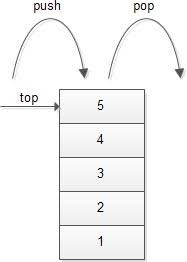# Maximum Equal Sum in Three Stacks

## Problem Statement

You are given three stacks with Non-negative elements in each of them. Your task is to find and return the maximum possible equal sum in the three stacks.

## Prerequisites

Before we think of the approach to tackle the above problem, it is necessary to know the stack data structure and its operations.

Stack is a LIFO( Last in, first out) type data structure. Meaning, the elements that are input last are retrieved first. Think of a stack of books. The last book in the stack is kept at the bottom and wouldn’t be retrieved until all the other books above it are removed.

Stack has three basic operations:-

• Push: The push operation inserts the element in the stack. The time complexity of the operation is O(1).
• Pop: The pop operation retrieves the last inserted element in the stack. The time complexity of the operation is O(1).
• Peek: The Peek or Top operation prints the last inserted element in the stack without removing it. The time complexity of the operation is O(1).

It’s seemingly simple to understand and implement as well as the operations are cheap. That is what makes it a powerful tool in problem-solving. Stack finds its uses in a number of scenarios, one of which we will study now.## Approach

Our task is to find the maximum possible equal sum in three stacks.

So let’s consider three stacks A, B, C with some elements-

A=[1, 3, 4, 2, 1, 6, 7]   {Initial Sum = 24}

B=[3, 2, 2, 1, 5, 9]       {Initial Sum = 22}

C=[5, 2, 1, 8]               {Initial Sum = 16}

Since we want to find the maximum possible equal sum in three stacks, we start by popping out elements from the stack with the maximum sum and then rechecking which stack has the maximum sum. We will repeat the process until the sum of all three stacks is equal. Though it’s important to observe, this approach can only work when all the elements in the stack are non-negative.

1. 7 is removed from A

Sum(A)=17

Sum(B)=22

Sum(C)=16

1. 9 is removed from B

Sum(A)=17

Sum(B)=13

Sum(C)=16

1. 6 is removed from A

Sum(A)=11

Sum(B)=13

Sum(C)=16

1. 8 is removed from C

Sum(A)=11

Sum(B)=13

Sum(C)=8

1. 8 is removed from C

Sum(A)=11

Sum(B)=13

Sum(C)=8

1. 5 is removed from B

Sum(A)=11

Sum(B)=8

Sum(C)=8

1. 1 is removed from A

Sum(A)=10

Sum(B)=8

Sum(C)=8

1. 2 is removed from A

Sum(A)=8

Sum(B)=8

Sum(C)=8

The loop stops when all the three sums reach a common value.

## Algorithm

1. Input non-negative elements for all three stacks.
2. Store the sums of all three stacks in three variables.
3. Check which stack has the maximum sum.
4. From the stack with the maximum sum, pop out the last element.
5. Subtract the last removed value from the sum of that stack.
6. Repeat steps 3 to 5 until all the three sums are equal.
7. Return the common value of the three sums.

## Complexity Analysis:

Time Complexity: In the worst case, we might have to iterate over all three stacks completely. So, if the size of each stack is N and we make 3N iterations, then the time complexity would be of the order O(N).

Space Complexity: We would require three stacks of size N to store the elements. Therefore, the space complexity is of the order O(N).

## FAQs

1. Briefly explain stack data structure.
The stack is a LIFO(last in, first out) type data structure. It has three basic operations- Push, Pop, Peek.

2. Brief about complexity analysis of the above algorithm.
Time Complexity: 3N iterations, so O(N).
Space Complexity: 3 stacks of N size, so O(N).

3. Can elements be inserted at any other position other than the top in a stack?
No, insertion of elements in a stack takes place only at the top of the stack.

4. What is the primary difference between a Stack and a Queue?
Stack- LIFO type data structure.
Queue- FIFO type data structure.

5. Mention some of the real-life applications of stack.
Undo/Redo commands work on the concept of a stack.
Back/Forward in browsers.

## Key Takeaways

Stack is one of the most important data structures as far as company interviews are concerned. Your knowledge and usage of stack is a very critical aspect of your interviews. Stacks are used extensively in our daily life applications and that’s what makes assessment of this data structure by companies almost necessary. We would highly recommend you to try more such questions on our coding platform Code Studio to solidify your concepts even further.

Happy Learning!